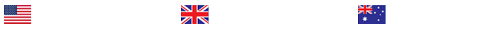compare measured and theoretical values of V, I and R in a series and parallel circuits using Kirchhoffs and Ohms laws.

Project description
all the necessary instructions and information will attach to the file section
Abstract and Introduction
A1) Abstract: A clearly stated aim for the experiment) (2), how aims were achieved (sub experiments summarised) (2) and what the final results were (2)
I1) Definition of voltage, current and resistance (3). Description of series and parallel circuits (4). Explanation of how voltage and current varies in series and parallel circuit (4). Description of Kirchhoffs 1st law (2) and 2nd law (2). Description Ohms law (2) including the equation (2).
6
19
Method
M1) Original correctly labelled (3) diagrams of the experimental set ups (3).
M2) An original account of each of the procedures written as a paragraph (4) and in the 3rd person and past tense (2).
6
6

Results

Series Circuits
R1a) Table of nominal resistance against measured resistance including % difference (2). (Calculations shown in Appendix). Table includes units (1) and correct value for total measured and calculated resistance (calculations shown in Appendix).
R2a) Table of measured value of V, I and R including % difference (2). (Calculations shown in Appendix). Table includes units (1) R1b) Correctly calculated values for the potential difference across each resistor. (Calculations using Ohms law shown in the appendix).
Parallel Circuits
R3) Table of measured value of V, I and R and calculated values for V, I and R (3). (Calculations shown in Appendix).

3
3
3
-1-
Foundation Engineering Science
Discussion
Conclusion
Series circuits
D1) Based on the results, comments on the relationship between V, I and R in a series circuit (3). Does this agree with the theory (2)?
D2) Discussion of the percentage difference between the total nominal resistances and total measured resistances (3). Explanation of the reasons for any difference between the total nominal resistances and total measured resistances (2).
D3) Comments on the value of the measured potential difference across each resistance compared to the calculated p.d across each resistor (3). Discussion of the reasons for any difference between the theoretical (calculated) values and measured values of VS and I in series circuit (4).
Parallel circuits
D4) Bases on the results, comment on the relation between the measured V and I in a parallel circuit (3). Does this agree with the theory (2)?
D5) Comments on the value of the total measured resistance compared to the calculated total resistance (3). Discussion of the reasons for any difference between the theoretical (calculated) values and measured values of V and I (4).
D6) Improvements to the method in order to gain a more accurate/precise result (4).
C1) A summary of what was found (6):

5
5
7
5
7
4 6

References and Appendix
Ref) Correct reference section (1). Correctly cited in text (1). App1) Calculations for table R1 (3). App2) Calculations for table R2 (4). App3) Calculations for table R3 (4).

13

Presentation

Pres) All word processed, graphs drawn in Excel, clearly structured (1).
2
Instruction files

_e2.pdf(730,88 KiB)
e2_marcking_scheme_.pdf(64,60 KiB)

Last Completed Projects

# topic title discipline academic level pages delivered
6
Writer's choice
University
2
1 hour 32 min
7
Wise Approach to
Philosophy
College
2
2 hours 19 min
8
1980's and 1990
History
College
3
2 hours 20 min
9
pick the best topic
Finance
School
2
2 hours 27 min
10
finance for leisure
Finance
University
12
2 hours 36 min